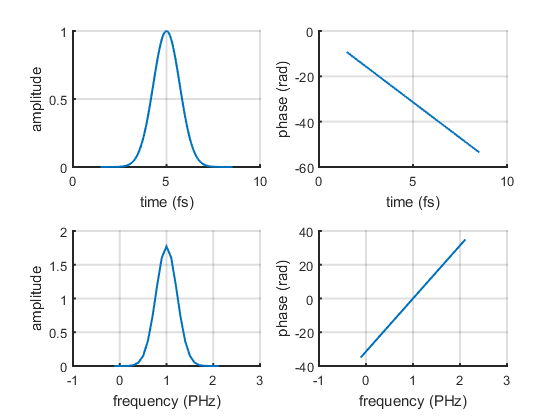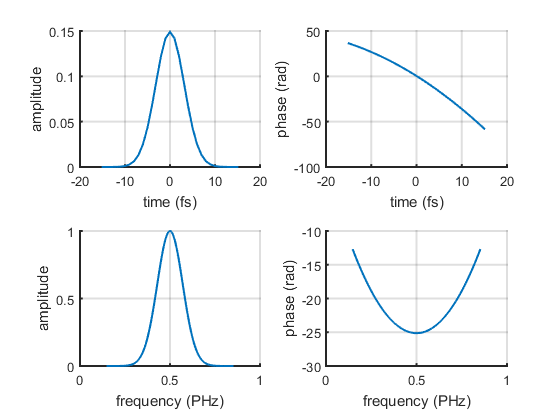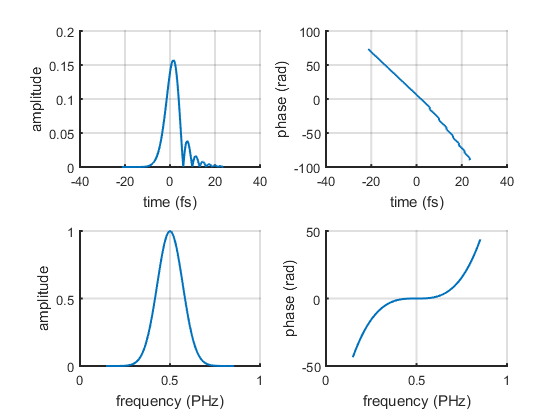# Examples of how to set-up a LaserPulse object

## New pulse in time domain

```% time array
t = linspace(0, 10, 1000);
% electric field
Et = exp(-(t-5).^2) .* exp(-2i*pi*t);
% LaserPulse object
p1 = LaserPulse(t, 'fs', Et);
% plot the pulse
p1.plot();
```## New pulse in frequency domain as complex field

```% frequency array
f = linspace(0, 1, 1000);
% electric field
Ef = exp(-(f-0.5).^2/(0.1)^2) .* exp(1i* 100*(f-0.5).^2);
% LaserPulse object
p2 = LaserPulse(f, 'PHz', Ef);
% plot the pulse
p2.plot();
```## New pulse in frequency domain as amplitude/phase pair

```% frequency array
f = linspace(0, 10, 1000);
% electric field
Af = exp(-(f-0.5).^2/(0.1)^2);
phi = 1000*(f-0.5).^3;
% LaserPulse object
p2 = LaserPulse(f, 'PHz', Af, phi);
% plot the pulse
p2.plot();
```